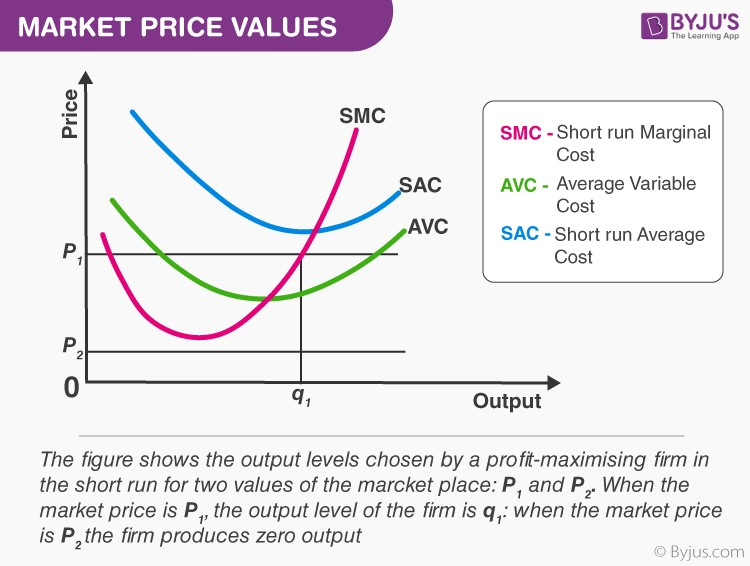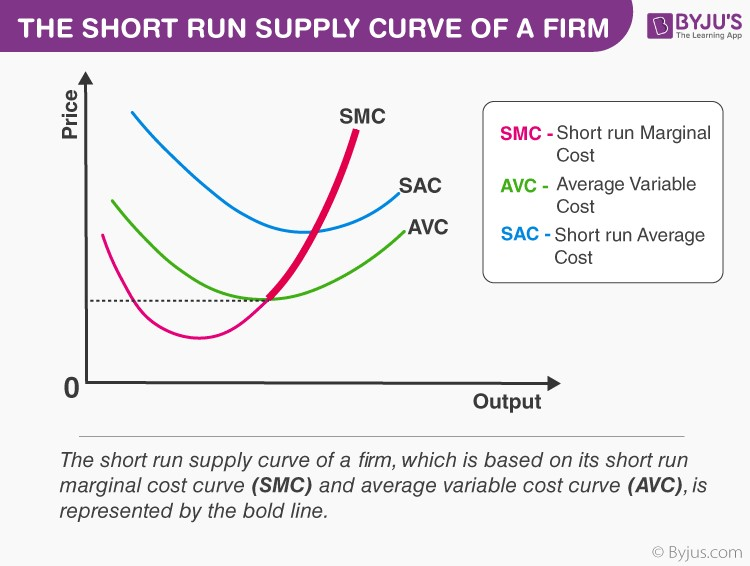# Short Run Supply Curve of a Firm

Let us derive a short-run supply curve for an enterprise. We shall divide this derivation into two parts. First, determine the enterprise’s profit-maximising output degree when the market cost price is greater than or equal to the minimum AVC. Now, we can determine the enterprise’s profit-maximising output degree when the market cost is less than the minimum AVC.

## Case 1: Price is greater than or equal to the minimum AVC

Assume that the market cost price is p1, which surpasses the minimum AVC. We begin by equalising p1 with SMC on the increasing part of the SMC curve; this leads to the output degree q1. Also, note that the AVC at q1 does not surpass the market cost price, p1. Hence, all the three conditions in section 3 are satisfied at q1. Therefore, when the market cost price is p1, the enterprises output degree in the short run is equal to q1.## Case 2: Price is less than the minimum AVC

Assume that the market cost price is p2, which is less than the minimum AVC. If a profit-maximising enterprise manufactures a positive output in the short run, then the market cost price, p2, must be greater than or equal to the AVC at that output degree. In the image, the AVC strictly surpasses p2. In other words, the enterprise cannot supply a positive output.  So, if the market price is p2, then the enterprise produces a zero output.